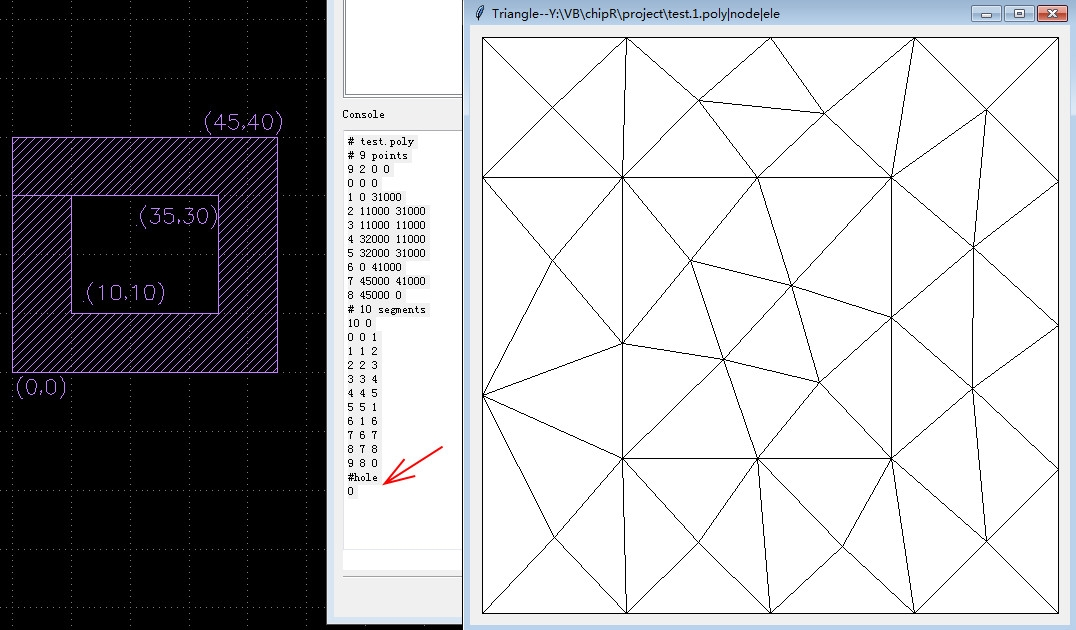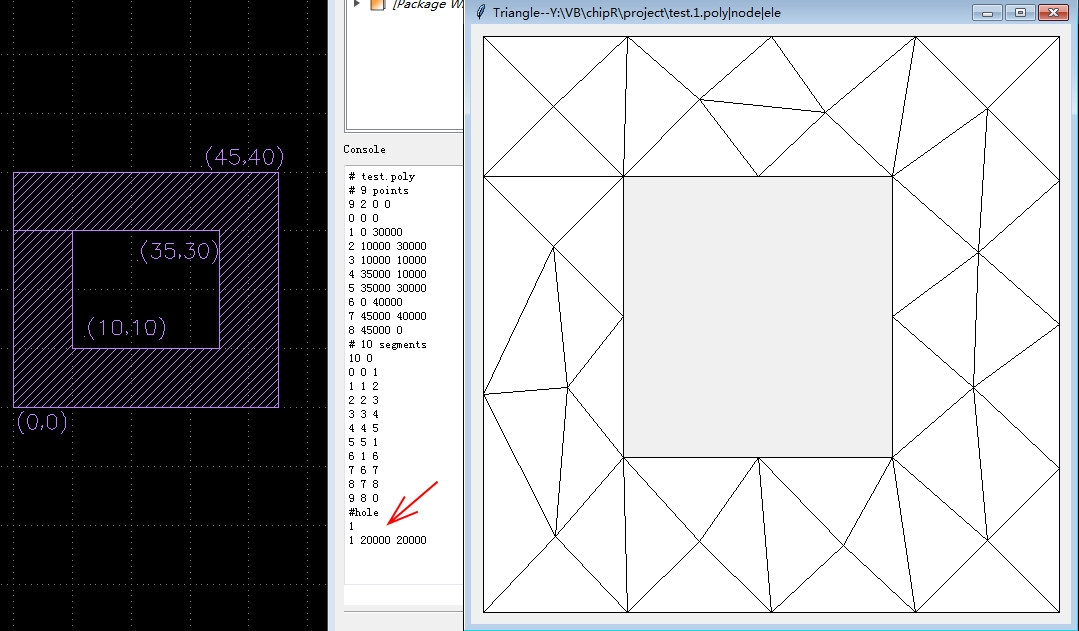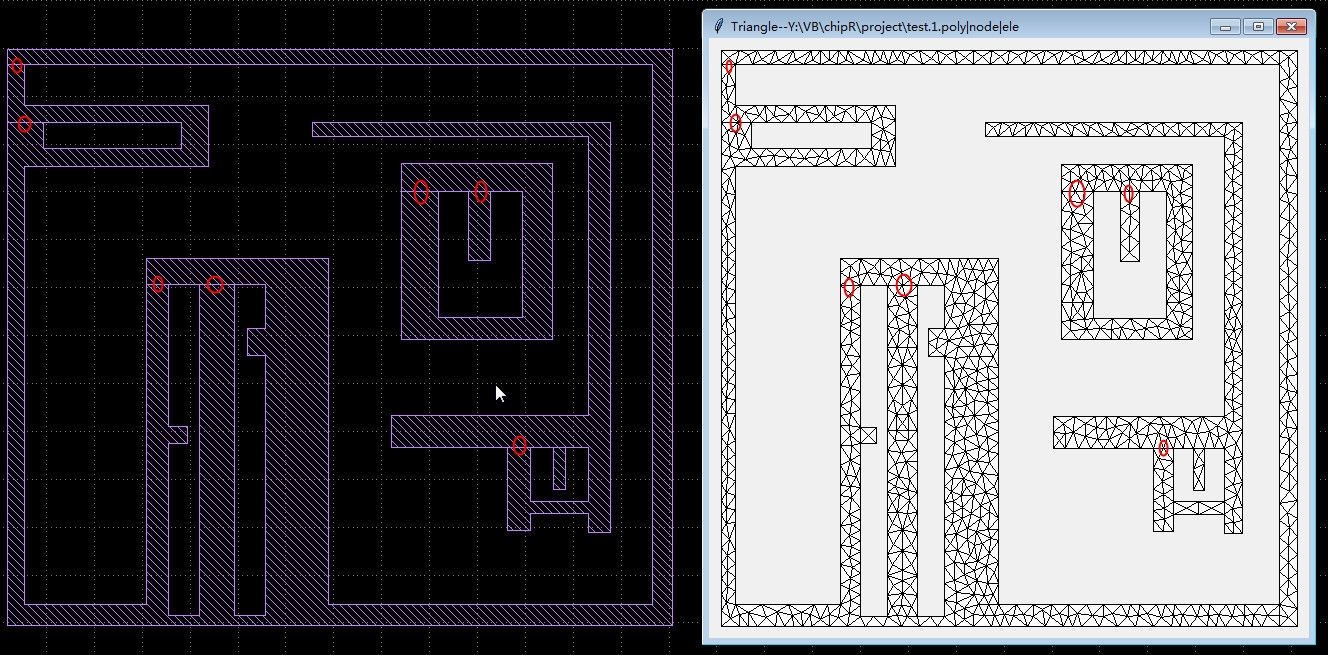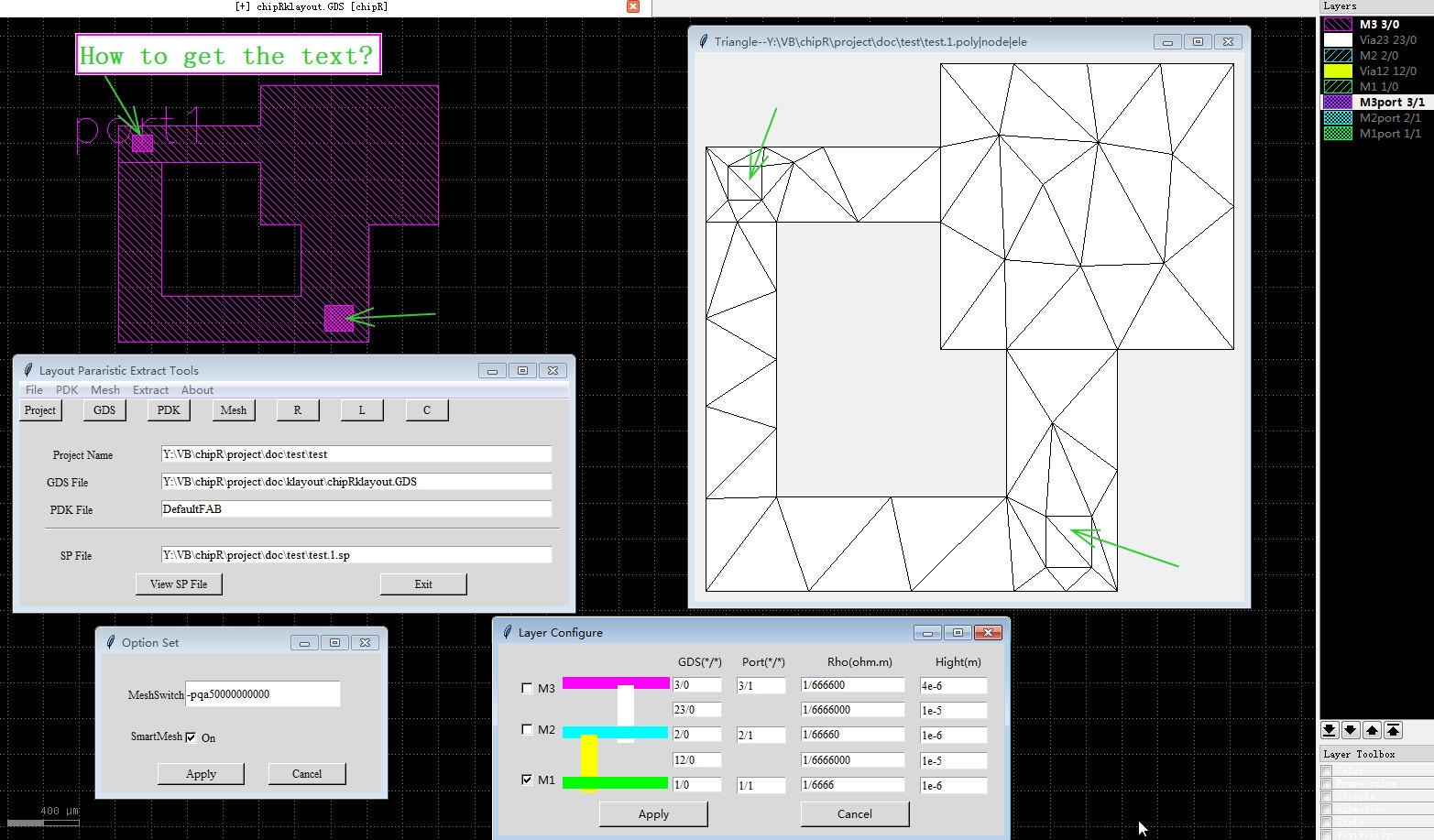# Creat Triangle poly file

Dear All,
I try to find a way to mesh one layout file.
1) export a .poly file from Klayout;
2) use Triangle to mesh this .poly file.

The .poly file represents a PSLG, as well as some additional information. PSLG stands for Planar Straight Line Graph, a term familiar to computational geometers. The detailed is in the link:
http://www.cs.cmu.edu/~quake/triangle.poly.html

I think the key is how to get the .poly file from Klayout.
Anyone can help me?
Or How do I create one python script to do this.

• Hi,

PSLG is what you get from KLayout's polygons. But there is no output to the .poly format yet. I don't think this is difficult. Here is a starting point. This script prints the hulls (outer contours) and holes of all polygons on a given layer:

``````import numpy as np
from scipy.spatial import Delaunay

cellview = pya.CellView.active()
ly = cellview.layout()
cell = cellview.cell

input_layer = ly.layer(2, 0)   # GDS layer 2, datatype 0

# fetch the input polygons and merge to make the polygons non-overlapping
# (specifically non-self-overlapping)
input_polygons = pya.Region(cell.begin_shapes_rec(input_layer))

for poly in input_polygons.each():

print("Hull")
for pt in poly.each_point_hull():
print(str(pt))

for h in range(0, poly.holes()):
print("Hole #" + str(h + 1))
for pt in poly.each_point_hole(h):
print(str(pt))
``````

Matthias

• Dear Matthias,
Thank you very much.
I will study every line of your code to familiar with Klayout's Python API.

I try to create one tool to extract one layout's parasitic RLC.
The tool is very like other tools such as Finite Element Analysis etc
The tool will
1)get .poly file,
2)then use Triangle.exe to mesh this .poly file, get many small triangle cells,
3)then extract RLC
I have finished step 2),3) by Python Code. The .poly file is the final step.
I write .poly file manually before.
Now I need one .poly file exported from Klayout automatically.

• # I finish the Code(but it just works, It is very slower than your original code):

``````# description: Export poly file to Triangle
# autorun

import numpy as np
#from scipy.spatial import Delaunay

cellview = pya.CellView.active()
ly = cellview.layout()
cell = cellview.cell

input_layer = ly.layer(2, 0)   # GDS layer 2, datatype 0
print("# test.poly")
# fetch the input polygons and merge to make the polygons non-overlapping
# (specifically non-self-overlapping)
input_polygons = pya.Region(cell.begin_shapes_rec(input_layer))

node=[] # all the vertices
segment=[] # all the segments

for poly in input_polygons.each():
# write the nodes of Hull #i
for pt in poly.each_point_hull():
if [pt] not in node:
node.append([pt])

# write the segments of Hull #i
for pt in poly.each_edge():
s1=str(node.index([pt.p1]))+' '+str(node.index([pt.p2]))
segment.append(s1)

# output node
print('# '+str(len(node))+' '+ 'points')
print(str(len(node))+' 2 0 0')
i=0
for n1 in node:
n1=str(n1)
n1=n1.replace(',',' ')
print(str(i)+' '+n1)
i=i+1

# output segment
print('# '+str(len(segment))+' '+ 'segments')
print(str(len(segment))+' 0')
i=0
for s1 in segment:
s1=str(s1)
s1=s1.replace(',',' ')
print(str(i)+' '+s1)
i=i+1

print('#hole')
print('0')
``````

1) select all shapes, then 'Edit-Selection-Merge Shapes';
2) Run this script;
3) get the right .poly file.

but I can not output the hole shapes.
(the hole line write one point which is any point in this hole)Add hole lines to show the holes

``````#hole
1    # only one hole
#  first hole, point(20000,20000) is inside this hole.
#  Holes are specified by identifying a (any) point inside each hole.
1 20000 20000
``````• How to code to output the hole?
Thanks.

• I got it.
All holes can be identified.
Thank Klayout's power functions!

``````hole=[] # all holes
for he in input_polygons.holes():
# write the nodes of Hull #i
h1=str(he.point_hull(0).x+1)+' '+str(he.point_hull(0).y+1)
hole.append(h1)
``````

so cool!
Below is my work. ( except the place where a red circle. But I can accept it)• Very goodThanks for sharing.

BTW: in the first post I forgot to delete the Delaunay import - I use it to check the decomposition. But I found that it only works on convex polygons. To me this looks like a too severe restriction as to be useful. Maybe I missing an important point here.

Regards,

Matthias

• Thanks to your great Klayout. I almost finish this tool.

I studied the Class Delaunay in scipy.spatial. It seems that the spatial's Delaunay is based on Qhull.exe. So I studied www.Qhull.org. Maybe, this Qhull is more powerfull than Triangle.exe. But I have no more time to study it, and The Triangle is a perfect tool to implement my work, I decided to use Triangle.exe still. Thank you for your Delaunay code, I learn another tool Qhull.

Only one remaining question:
How to get the text in one layer ( for example, 2/0 )?

In my tool,
Layer(2,0)=metal2;
Box in Layer(2,1)=port;
Text in Layer(2,1)=port's name
So I must get this Text to identify every port.

This Code can not get text.

``````cellview = pya.CellView.active()
ly = cellview.layout()
cell = cellview.cell
input_layer = ly.layer(3, 1)   # GDS layer 3, datatype 1 for Port's shape  and Port's Text
print(cell.shapes(input_layer).STexts)
``````

Thanks.

BTW, Below is the tool GUI:• You can iterate the texts this way:

``````for s in cell.shapes(input_layer).each(pya.Shapes.STexts):
position = s.bbox().center()
text = s.text_string
print("position: " + str(position) + ", text: " + text)
``````

Regards,

Matthias

• Thank you,
I finished my work.
I am not a programmer. To implement this tool I studied Klayout's API by myself. It is a hard work for me.
So thank you for your help.
Now the tool works well.

It extracts the layout's parasitic parameter up to 3 layers(M3/Via2/M2/Via1/M1) now.
Of course, It can deal with any numbers of layer.

It extracts the parasitic value very fast.

Now I use the macro to export mesh file.
The future work is to use python's pya to export it.
If using pya, the tools do not need to open the klayout.

Thank you again.